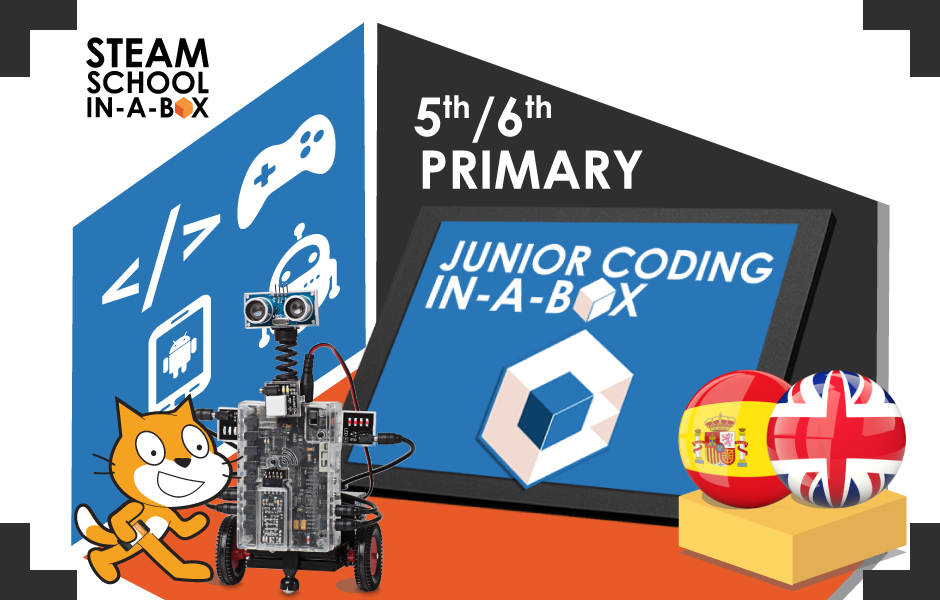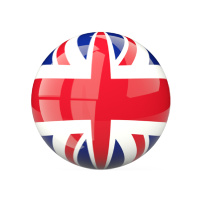### 5TH & 6TH PRIMARY: PROGRAMMING AND ELECTRO-ROBOTICSSCHOOL STAGE: Primary (5th/6th)

LANGUAGES:ESTIMATED TEACHING HOURS: 58

TECHNOLOGIES: Scratch, Arduino

CURRICULAR AND KEY COMPETENCES:
BOCM: DECRETO 89/2014 (PÁG. 47)

COURSE ACCREDITATION:

In the full course they will be getting badges as the reach the objectives of the course.

### DESCRIPTION

Students will work on mathematical concepts of the third cycle of Primary through programming and electronics with Scratch and Arduino.

Through fun projects such as “Snake” or “Analog Clock”, they will learn to use important programming elements such as conditionals, variables and messages. At the same time they will also learn mathematical skills such as fractions, mental calculation, time measurement or geometry.

In addition, they will expand programming knowledge by starting in electronics with Arduino, making simple real circuits and using components such as breadboards, LEDs and push buttons.

### MATHEMATICAL CURRICULAR COMPETENCES

• Read, write and compare natural numbers up to 999
• Uses and automates the standard division algorithm
• Visually recognises the correspondence between a fraction and its graphic representation
• Identifies the time on analogue clocks and the equivalence between minutes and seconds
• Properly use turns and angles for the movement of clock handles
• Describes and locates the situation of an object using spatial concepts
• It identifies, classifies and describes quadrilaterals and triangular geometric forms, using the appropriate geometric vocabulary
• Recognize the differences between a circle and a circumference
• Knows and uses angles to design geometric shapes
• Correctly sorts random and non-random events

### TECHNOLOGICAL AIMS

• PROGRAMMING OF VIDEO GAMES ORIENTED TO THE MATHEMATICS
• Easily use the different basic tools of the programming environment
• Places and moves objects in a given direction
• Starts and stops running a program
• Handles with ease the main groups of blocks in the environment
• It analyses the operation of a program from its blocks
• It modifies, through editing, the appearance of objects. Create new objects: actors, backgrounds and sounds
• Properly uses messages, variables and lists
• Use costumes
• Working with conditionals
• Use comparative and mathematical operators correctly
• Use mathematical operations
• Understand how loops work
• Draw with the pencil tool
• Use keyboard events
• Manage interaction between different objects
• Manage interaction between different objects by sending and receiving messages
• Programmatically Create Interactive User Questions and Answers
• ROBOTICS
• Understand how block programming works with Ardublock
• Differentiate electronic devices: actuators, sensors and others
• Learn the concept of variable
• Working with conditionals
• Use different types of operators
• Understanding and controlling servos